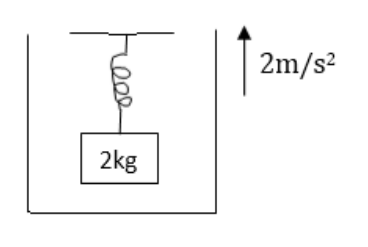# Solve the following :

Question:

Suppose the ceiling in the previous problem is that of an elevator which is going up with an acceleration of $2.0 \mathrm{~m} / \mathrm{s}^{2}$. Find the elongations.Solution:

$-k x=2\left(a_{0}+g\right)$

$=2(2+g)$

$x=\frac{-2(2+g)}{k}=\frac{-2(2+9.8)}{100}=-0.236 \mathrm{~m}$

$-k x^{\prime}=3\left(a_{0}+g\right)$

$=3(2+g)$

$x^{\prime}=\frac{-3(2+g)}{k}=\frac{-3(2+9.8)}{100}=-0.36 m$

$x^{\prime}-x=0.36-0.24 m=0.12 m$